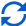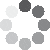Enter parameters Plate calculator
Plywood calculator
Length:
in
• inches (in)
• feet (ft)
• yards (yd)
• millimeters (mm)
• centimeters (cm)
• meters (m)
Width:
in
• inches (in)
• feet (ft)
• yards (yd)
• millimeters (mm)
• centimeters (cm)
• meters (m)
Thickness:
in
• inches (in)
• feet (ft)
• yards (yd)
• millimeters (mm)
• centimeters (cm)
• meters (m)
Metal:
Steel
• Steel
• Aluminium
• Berilium
• Brass
• Cast Iron
• Copper
• Gold
• Molybdenym
• Nickel
• Silver
• Stainless S300
• Stainless S400
• Titanium
• Zinc
• Zirconium
Quantity:
Price per:
Sheet
• Sheet
• pounds (lb)
• US tons (US t)
• tons (t)
• kilograms (kg)
• grams (g)
• milligrams (mg)
• ounces (oz)
CalculateResult
Total Weight:
0
lb
• pounds (lb)
• US tons (US t)
• tons (t)
• kilograms (kg)
• grams (g)
• milligrams (mg)
• ounces (oz)
One Plate:
0
lb
• pounds (lb)
• US tons (US t)
• tons (t)
• kilograms (kg)
• grams (g)
• milligrams (mg)
• ounces (oz)
Cost:
0(No Ratings Yet)Loading...
Real-time graphics. Make the calculations and see the changes.
Embed this calculator on your site!Add live graphics
Copied to clipboard! PreviewWhat's wrong?
Minimum 5 characters!